# How to interpret a passage in Purcell's Electricity and Magnetism

• zenterix
zenterix
Homework Statement
While reading Chapter 1 of Purcell's Electricity and Magnetism, I noticed there are various paragraphs where he attempts to make an intuitive argument to explain a concept, result, or equation. Here is an example that I can't understand.

"The field of an infinitely long line charge, we found, varies inversely as the distance from the line, while the field of an infinite sheet has the same strength at all distances. These are simple consequences of the fact that the field of a point charge varies as the inverse square of the distance. If that doesn’t yet seem compellingly obvious, look at it this way: roughly speaking, the part of the line charge that is mainly responsible for the field at P in Fig. 1.24 is the near part – the charge within a distance of order of magnitude r. If we lump all this together and forget the rest, we have a concentrated charge of magnitude ##q \approx \lambda r##, which ought to produce a field proportional to ##\frac{q}{r^2}## or ##\frac{\lambda}{r}##. In the case of the sheet, the amount of charge that is “effective,” in this sense, increases proportionally to ##r^2## as we go out from the sheet, which just offsets the ##\frac{1}{r^2}## decrease in the field from any given element of charge.
Relevant Equations
The confusing part for me starts at "roughly speaking".

The "near" part of the line: is that a point or a section of the line?

What are we "lumping" together exactly? ##\lambda r## seems like the charge that we'd have if the charge were distributed along the line from point ##P## to the line charge.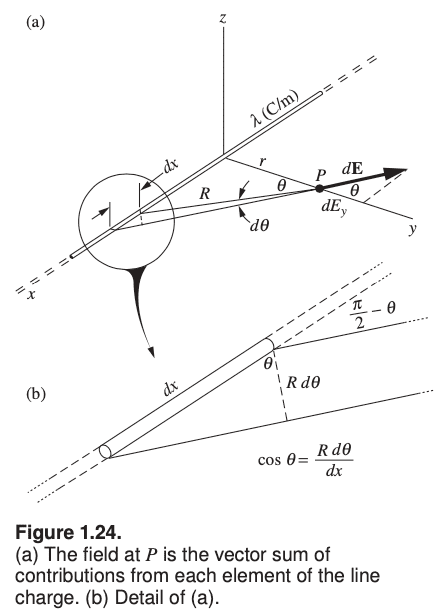Homework Helper
Gold Member
Relevant Equations:: The confusing part for me starts at "roughly speaking".

The "near" part of the line: is that a point or a section of the line?
The near part is a section of the line charge of length roughly equal to the distance r from the line charge to point P.

Imagine the line of charge broken into very small, equal-sized segments. Each segment is roughly a point charge.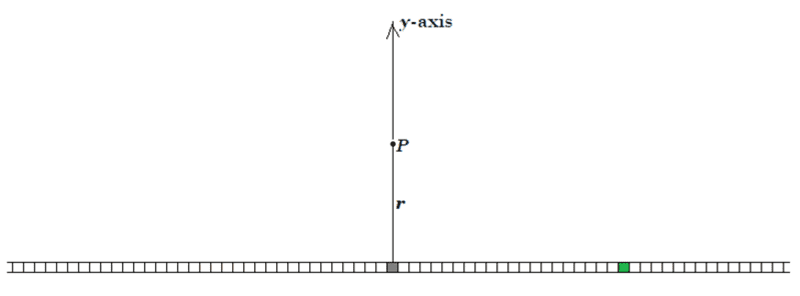The small segment that contributes the most to the field at P is the segment at the origin (directly below P), shown in grey. This segment contributes the most for two reasons: (1) It's the segment closest to P. (2) The electric field from this segment at P is entirely along the y-axis.

Segments close to the grey segment will also contribute a lot to the field at P.

If you take a segment of the line charge that is relatively far away, such as the green segment, it will not contribute nearly as much to the electric field at P since it is quite a bit farther away from P and the field of a point charge falls off as the square of the distance. Also, the field from the green segment at P points in a direction at a considerable angle to the y-axis. So, its y-component will be relatively small.

So, Purcell suggests that you can get a fair approximation to the field at P by neglecting segments of the line charge that are "far" from the origin. This might not be obvious since there are infinitely many segments that are "far" from the origin and only a finite number of segments "near" the origin. However, because of the two factors mentioned above (inverse square behavior and increasing angle of E relative to the y-axis for segments far away), this hand-waving turns out to be OK.

[I don't think this type of hand-waving would work for finding the electric potential V at P. You might not have studied electric potential yet. That's chapter 2.]

It also seems reasonable that the transition from "near" to "far" occurs roughly for segments whose distances from the origin are of the same order of magnitude as the distance ##r## between the line charge and P. The "near" segments would be roughly the shaded segments shown below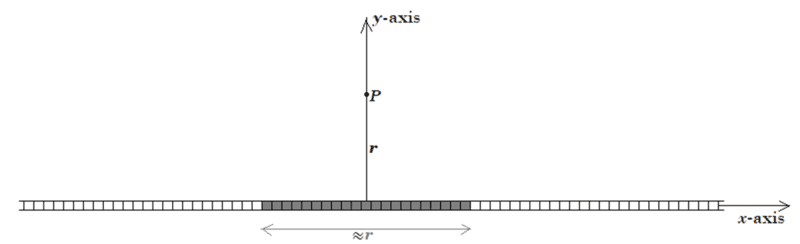It doesn't really matter if you take the length of the shaded region to be ##r## or ##2r## or any other length of order of magnitude ##r##. The shaded region is what Purcell is calling the "near part" of the line charge.

What are we "lumping" together exactly? ##\lambda r## seems like the charge that we'd have if the charge were distributed along the line from point ##P## to the line charge.
##\lambda r## is the order of magnitude of the charge in the shaded region; i.e., it's the charge in the "near part".

•Delta2, marcusl and zenterix
zenterix
Interesting, and makes sense now. I see a lot of geometric arguments used so far to discuss fields - electric and gravitational so far. There are a lot of corollaries to the inverse ##r^2## relationship of distance and field.

I am finding it eery how many of these passages there are that aren't difficult technically, but just difficult to understand, not sure if it's the author or what. Here is another example: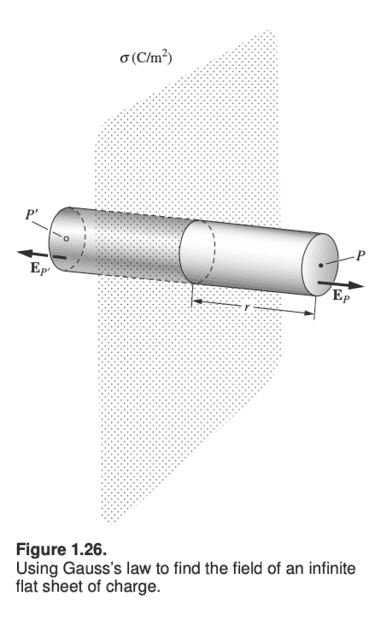Section 1.13 quickly derives the field of an infinite flat sheet of charge.

$$E_p = \frac{\sigma}{2\epsilon_0}$$ Then the text says

"In the more general case where there are other charges in the vicinity, the field need not be perpendicular to the sheet, or symmetric on either side of it. Consider a very squat Gaussian surface, with ##P## and ##P′## infinitesimally close to the sheet, instead of the elongated surface in Fig. 1.26. We can then ignore the negligible flux through the cylindrical “side” of the pillbox, so the above reasoning gives ##E_{\perp,P}+E_{\perp,P'}=\frac{\sigma}{\epsilon_0}##, where the ##\perp## denotes the component perpendicular to the sheet. If you want to write this in terms of vectors, it becomes ##\vec{E}_{\perp,P}-\vec{E}_{\perp,P'}=\frac{\sigma}{\epsilon_0}\vec{n}##, where ##\vec{n}## is the unit vector perpendicular to the sheet, in direction of ##P##. In other words, the discontinuity in ##\vec{E}_{\perp}## across the sheet is given by

$$\Delta \vec{E}_{\perp}=\frac{\sigma}{\epsilon_0}\hat{n}\tag{1.41}$$

Only the normal component is discontinuous; the parallel component is continuous across the sheet. So we can just as well replace the ##\Delta \vec{E}_{\perp}## in Eq. (1.41) with ##\Delta \vec{E}##."

My understanding here is that the author is skipping some parts of equations because he is automatically simplifying them. This is how I depict the case of a very "squat" gaussian surface (red) around the flat sheet (green):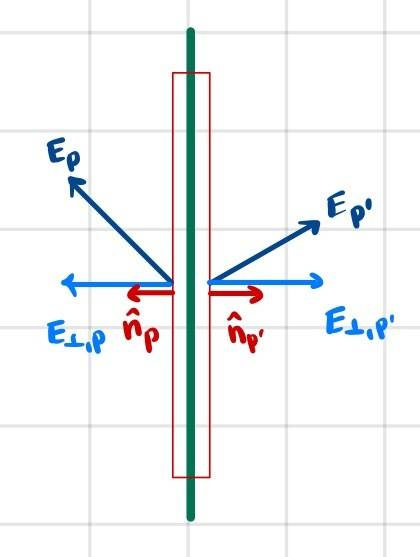Gauss's Law
##P## and ##P'## are infinitesimally close to the sheet, so the cylindrical part of the pillbox above is so small that we neglect the flux through it, and only consider the end-caps of the cylinder.

$$\vec{E}_{\perp,P}\cdot \hat{n}_P+\vec{E}_{\perp,P'} \cdot \hat{n}_{P'}=\frac{\sigma A}{\epsilon_0}\tag{1}$$
$$\implies E_{\perp,P}+E_{\perp,P'}=\frac{\sigma}{\epsilon_0}$$

To get the relationship in the text, namely
$$\vec{E}_{\perp,P}-\vec{E}_{\perp,P'}=\frac{\sigma}{\epsilon_0}\vec{n}$$

I guess you would multiply ##(1)## by ##\hat{n}_P## on both sides.

The discontinuity in the electric field vector across the sheet is thus ##\frac{\sigma}{\epsilon_0}\hat{n}_P##

If ##\vec{E}_{\perp,P'}=-\vec{E}_{\perp,P}##, which is the case for a flat sheet, then we simply get a vector form of our previous result for the electric field of a flat sheet

$$\vec{E}_P=\frac{\sigma}{2\epsilon_0}\hat{n}_P$$

Okay, so looks like I actually now understood all the steps by writing them down here. The result seems to be that when we model something as a flat sheet of charge, the difference in the electric field across the sheet (ie, the discontinuity in ##\vec{E}##) is ##\frac{\sigma}{\epsilon_0}\hat{n}##

Even if the sheet isn't infinite and we actually have an electric field component parallel to the sheet, the difference in the electric field vectors immediately to one side and immediately to the other is still a vector that is perpendicular to the sheet.

Last edited:
•Delta2
Homework Helper
Gold Member
Gauss's Law
$$\vec{E}_{\perp,P}\cdot \hat{n}_P+\vec{E}_{\perp,P'} \cdot \hat{n}_{P'}=\frac{\sigma A}{\epsilon_0}\tag{1}$$
$$\implies E_{\perp,P}+E_{\perp,P'}=\frac{\sigma}{\epsilon_0}$$
OK, except you left out the area ##A## in the two terms on the left side of the first equation.

To get the relationship in the text, namely
$$\vec{E}_{\perp,P}-\vec{E}_{\perp,P'}=\frac{\sigma}{\epsilon_0}\vec{n}$$

I guess you would multiply ##(1)## by ##\hat{n}_P## on both sides.
Yes, you can multiply ##E_{\perp,P}+E_{\perp,P'}=\frac{\sigma}{\epsilon_0}## by ##\hat n_P## to get $$E_{\perp,P} \, \hat n_P+E_{\perp,P'} \, \hat n_P=\frac{\sigma}{\epsilon_0} \hat n_P$$.

Then note that ##E_{\perp,P} \, \hat n_P = \vec{E}_{\perp,P}## and ## E_{\perp,P'} \, \hat n_P = -\vec{E}_{\perp,P'}##

The discontinuity in the electric field vector across the sheet is thus ##\frac{\sigma}{\epsilon_0}\hat{n}_P##
Yes

If ##\vec{E}_{\perp,P'}=-\vec{E}_{\perp,P}##, which is the case for a flat sheet, then we simply get a vector form of our previous result for the electric field of a flat sheet

$$\vec{E}_P=\frac{\sigma}{2\epsilon_0}\hat{n}_P$$

Okay, so looks like I actually now understood all the steps by writing them down here, though I did not really feel that it gave me any insight. This is all basically saying that even if the sheet isn't infinite and we actually have an electric field component parallel to the sheet, the difference in the electric field vectors immediately to one side and immediately to the other is still a vector that is perpendicular to the sheet.
Yes

•zenterix
•